Physics Numericals Class 11 Chapter 8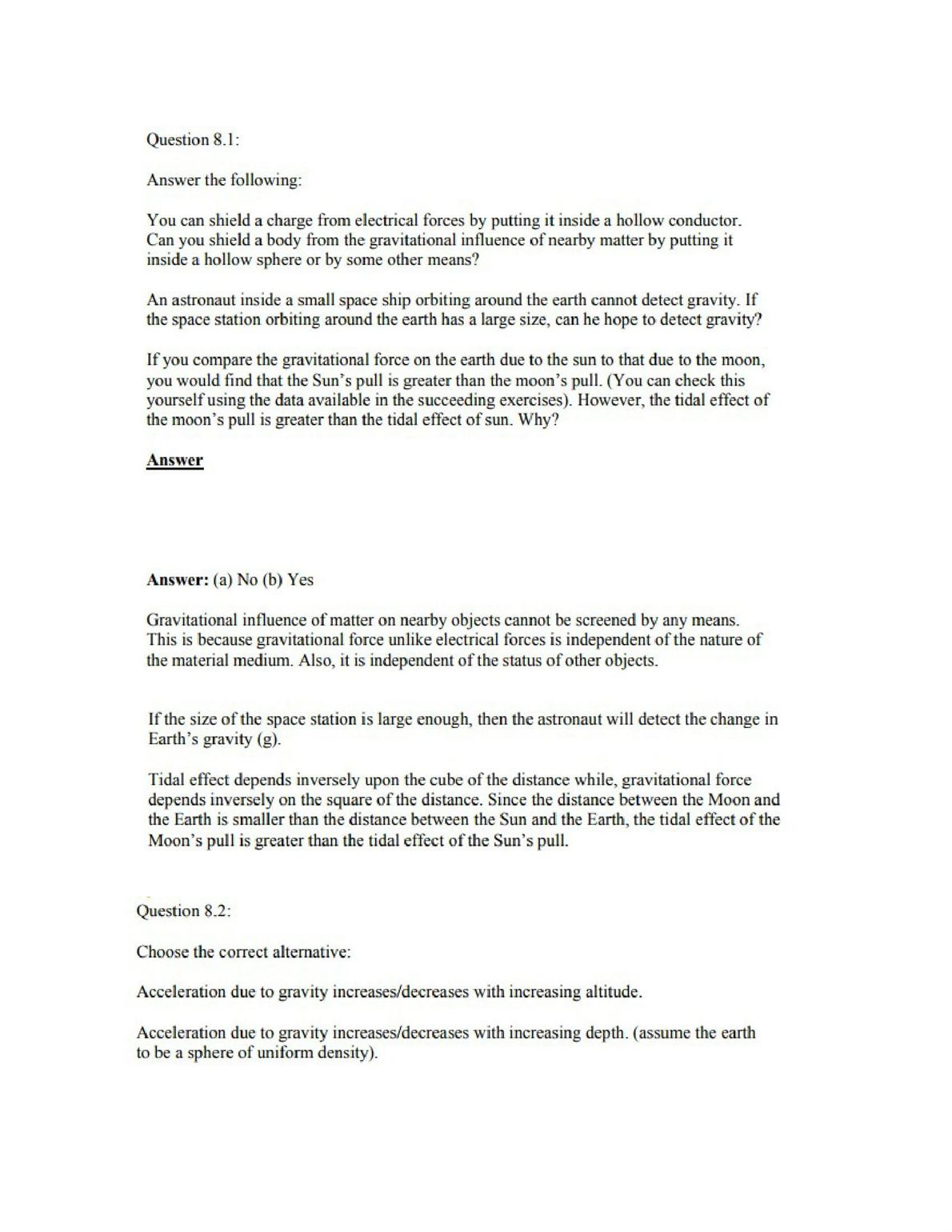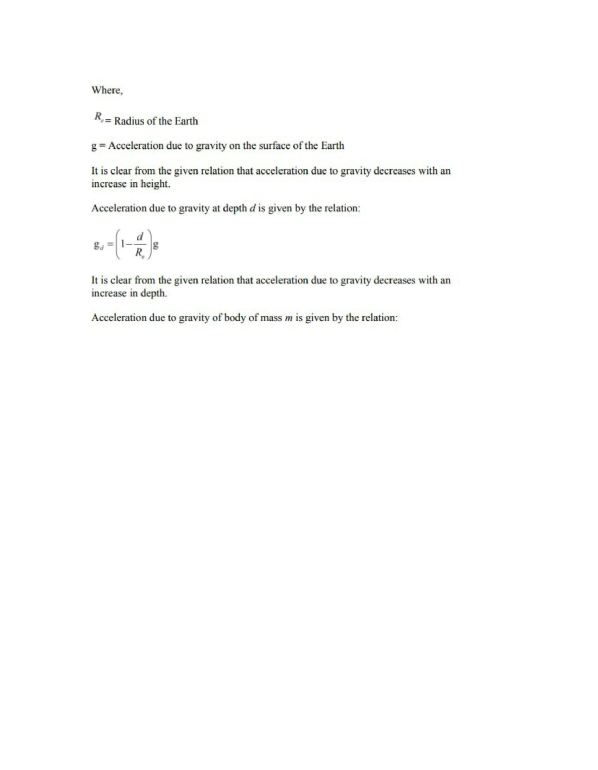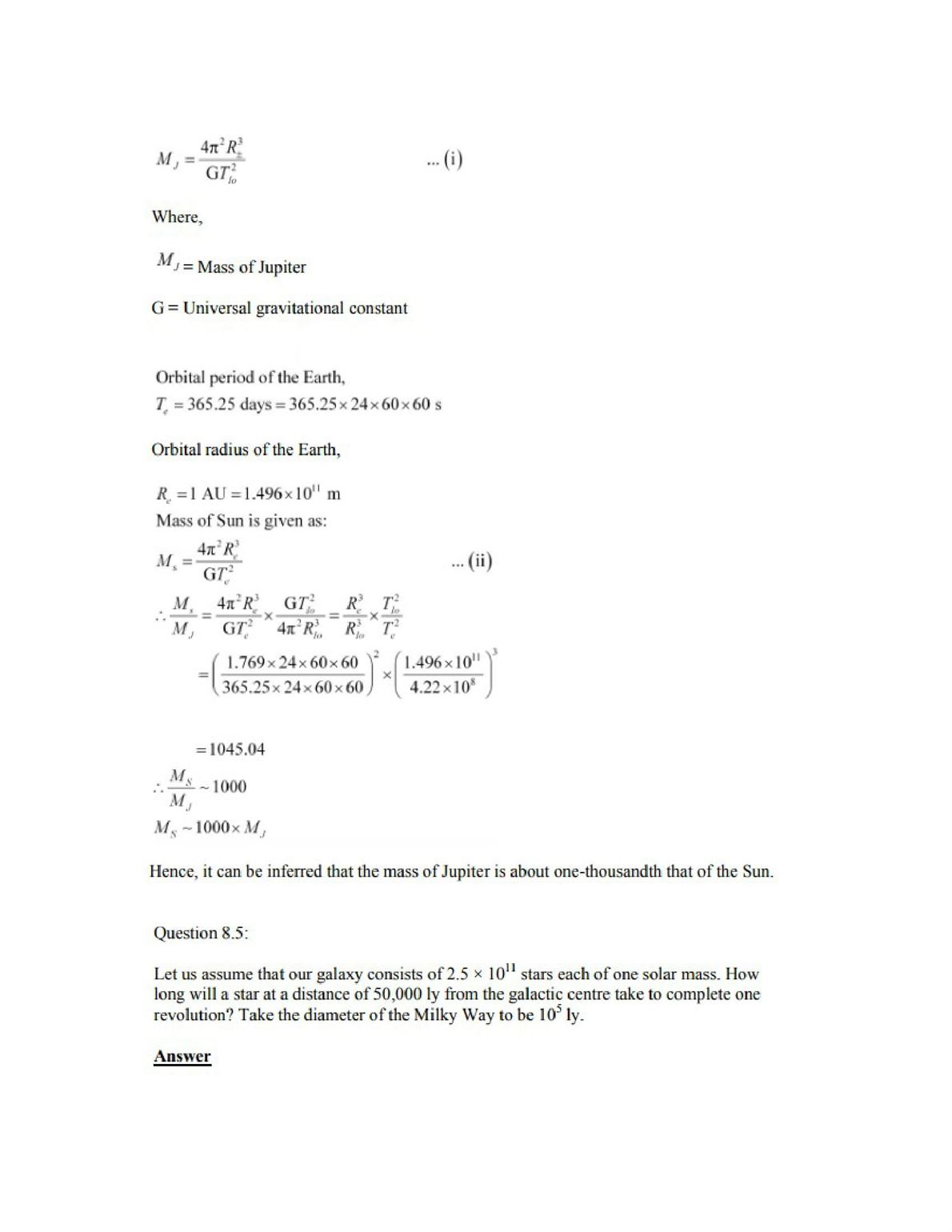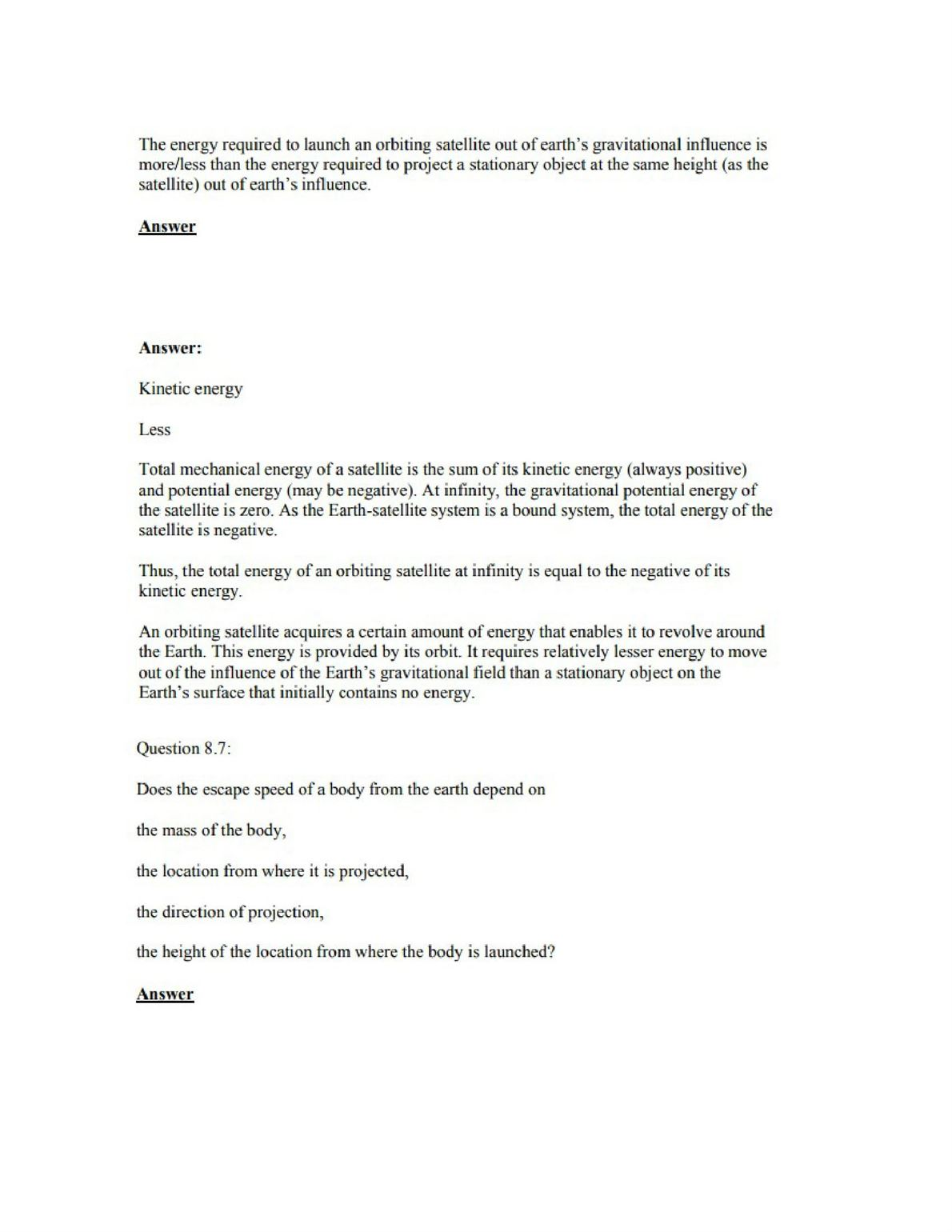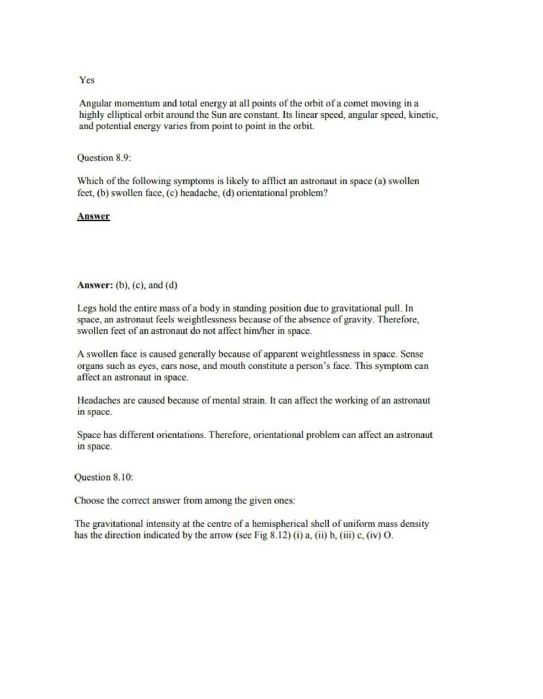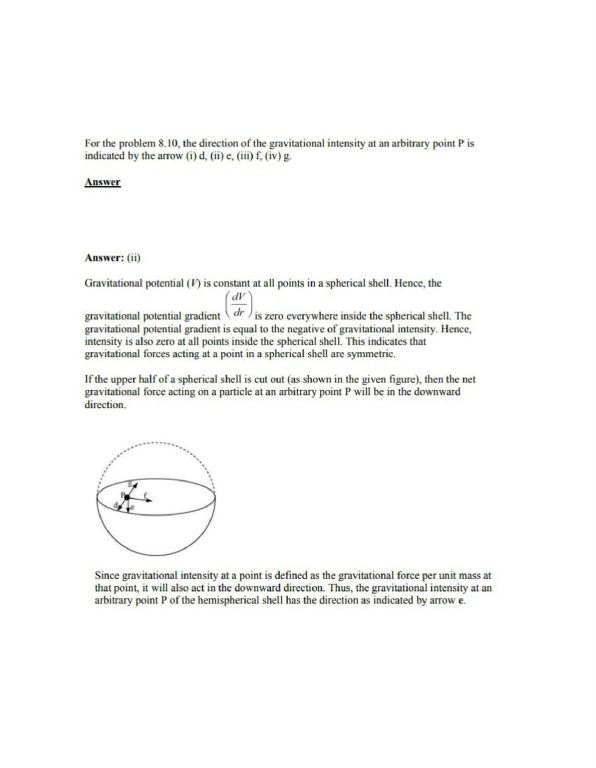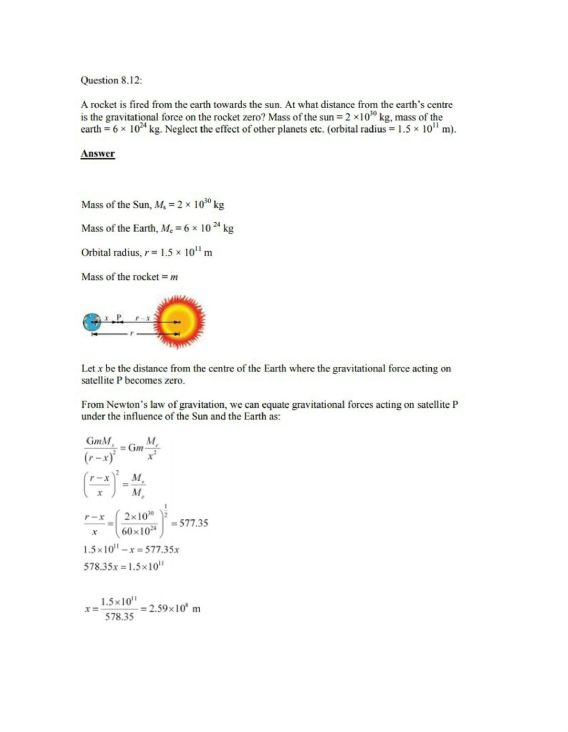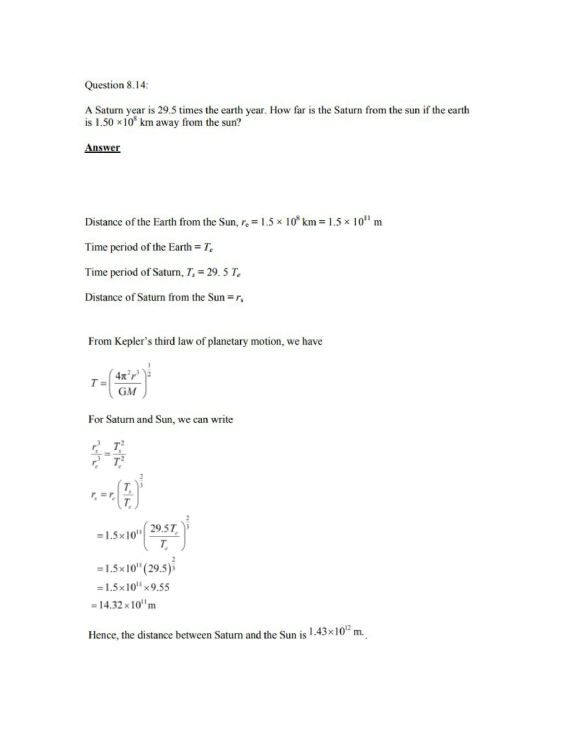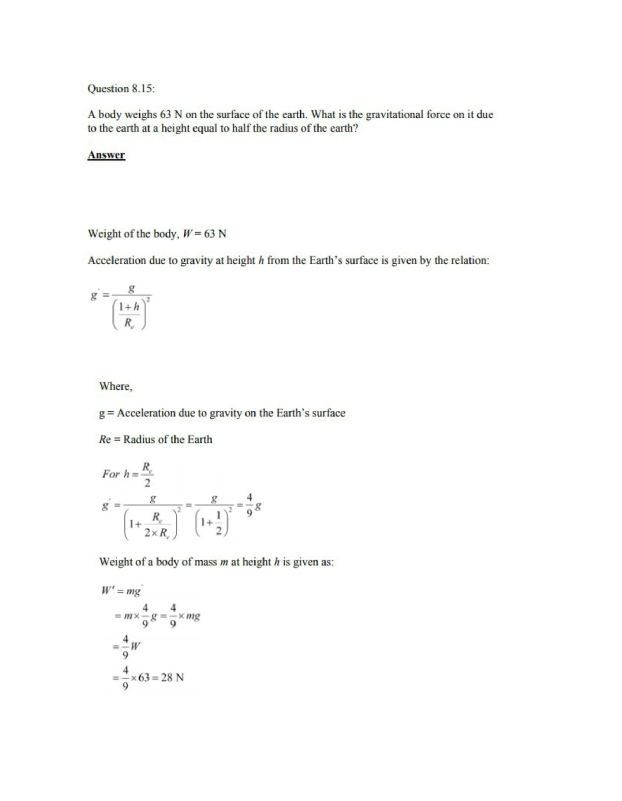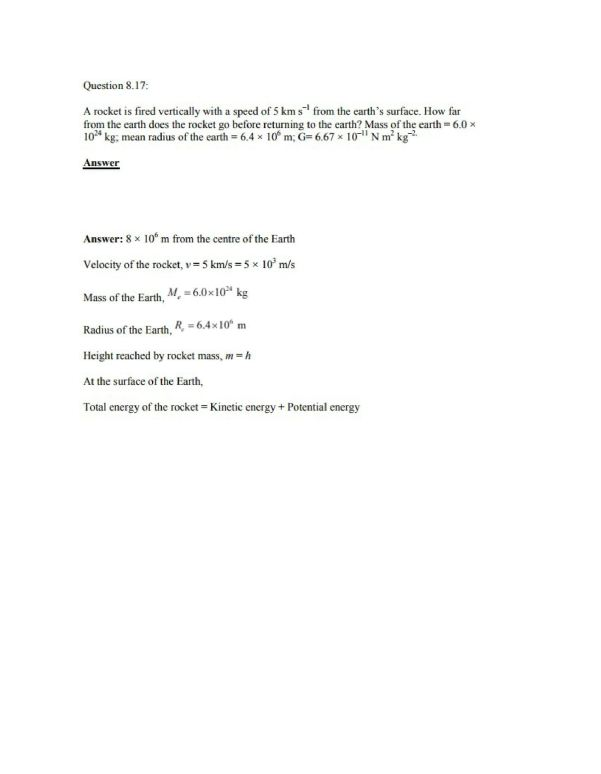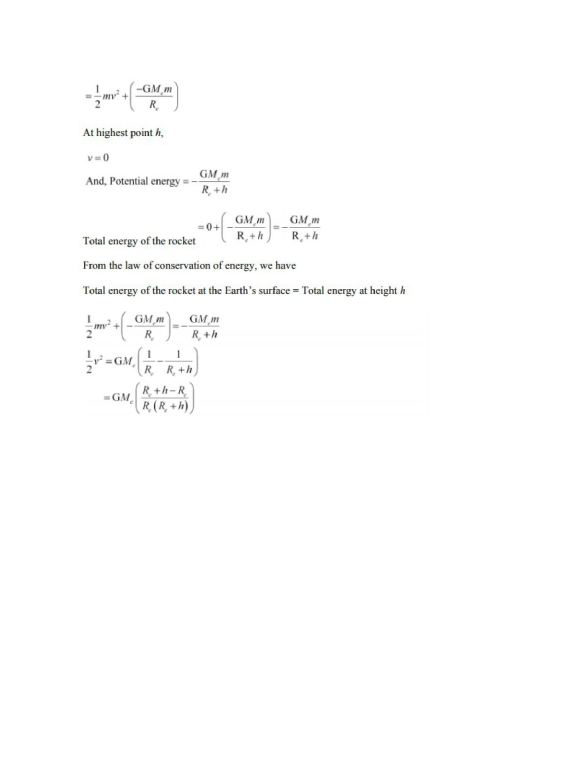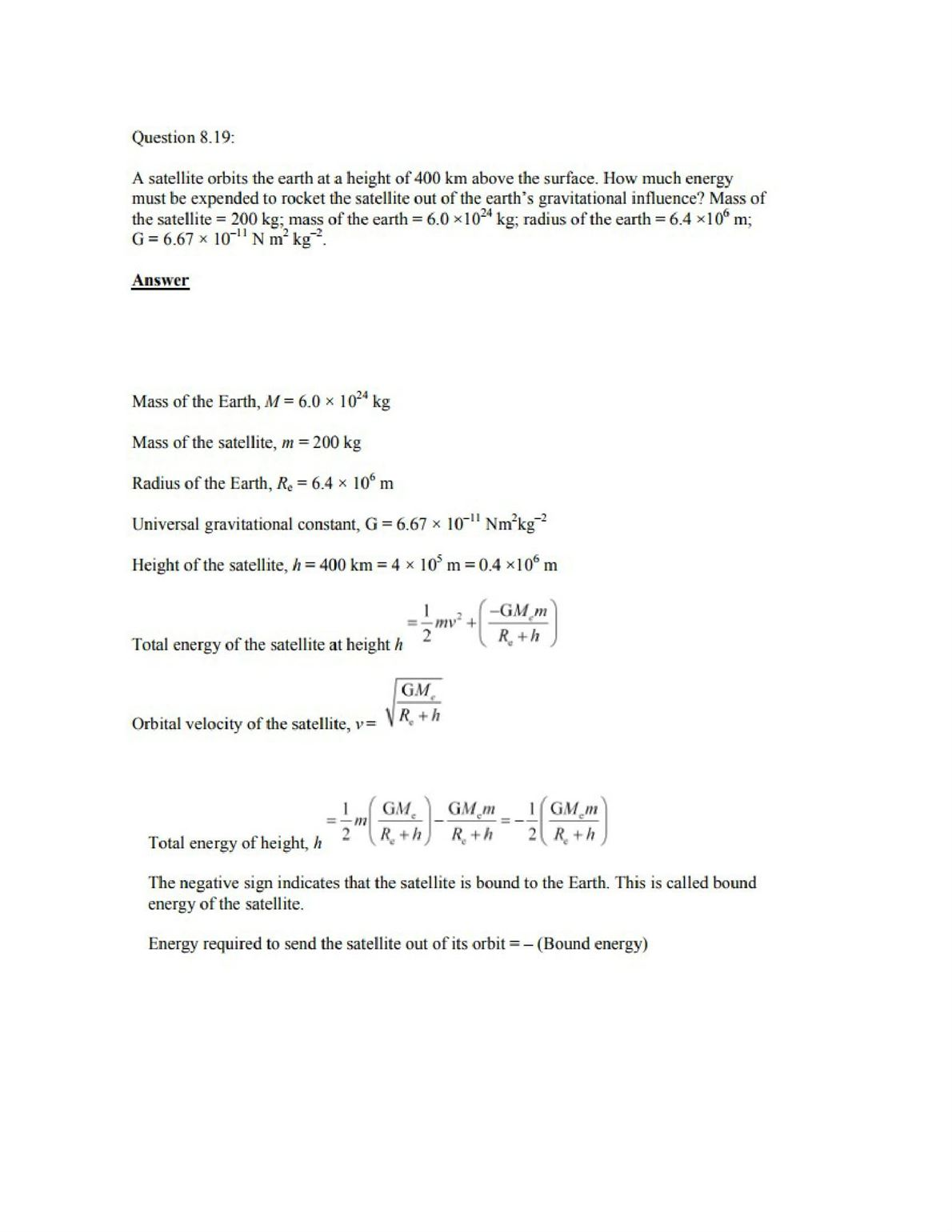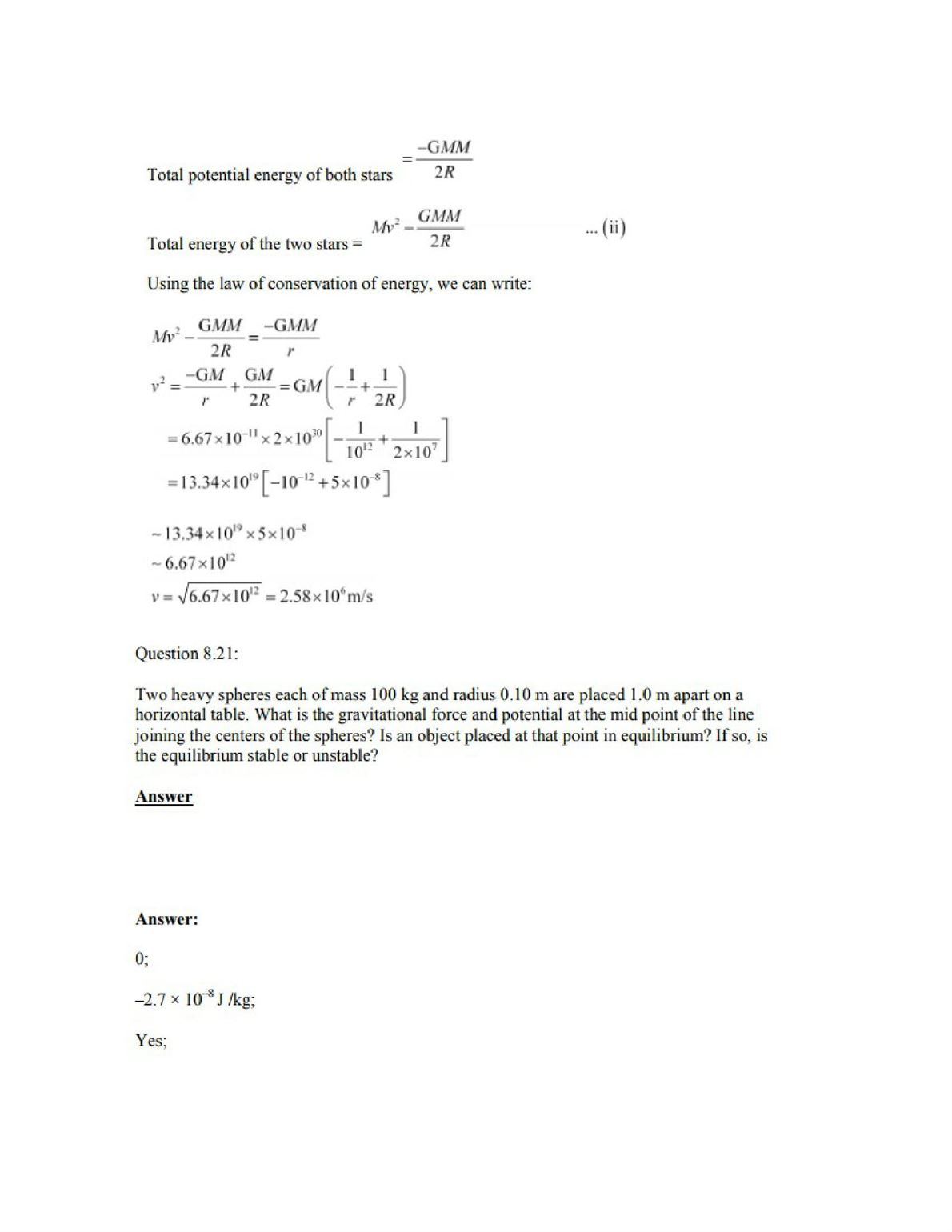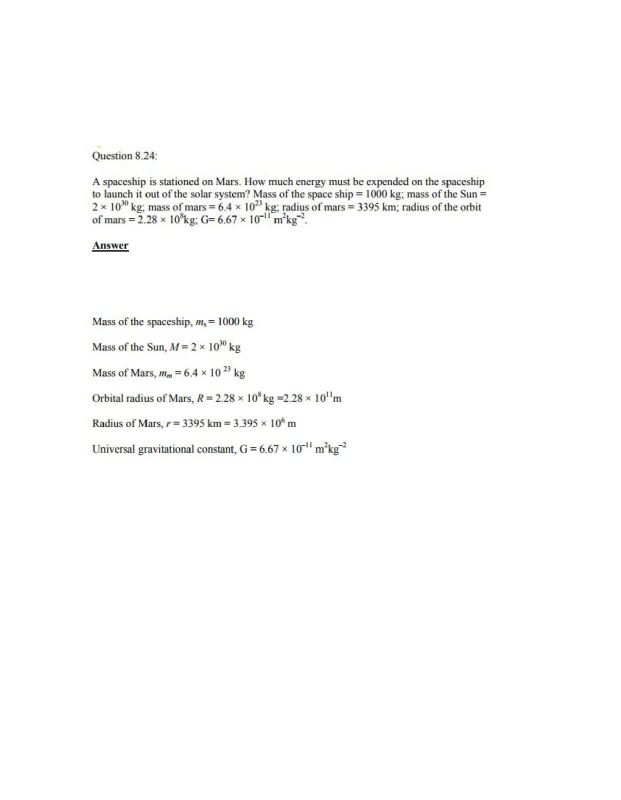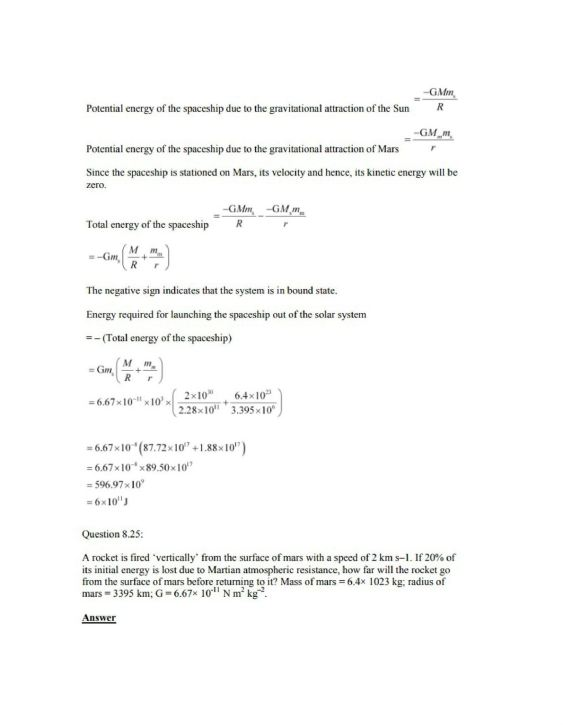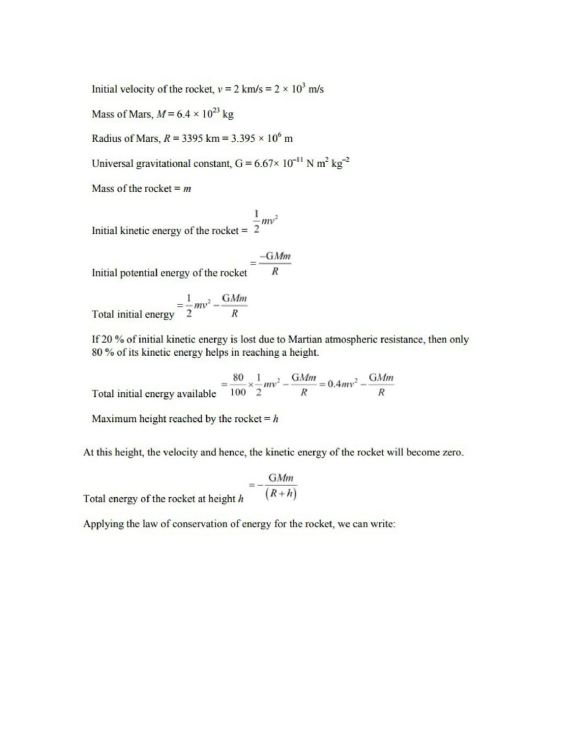Practise This Question

A body of mass 1 kg is executing simple harmonic motion.  Its displacement x (in cm) at time t (in second) is given by
x=6 sin(100 t+π4)The maximum kinetic energy of the body is